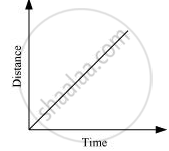CBSE Class 7CBSE
Share

Show the Shape of the Distance-time Graph for the Motion in the Following Cases: a Car Moving with a Constant Speed. - CBSE Class 7 - Science

ConceptDistance-Time Graph

Question

Show the shape of the distance-time graph for the motion in the following cases:

A car moving with a constant speed.

Solution

A car moving with a constant speed covers equal distance in equal intervals of time.

Such motion of car is represented in the given distance-time graph.Is there an error in this question or solution?

APPEARS IN

NCERT Solution for Science Textbook for Class 7 (2018 to Current)
Chapter 13: Motion and Time
Q: 7.1 | Page no. 156
Solution Show the Shape of the Distance-time Graph for the Motion in the Following Cases: a Car Moving with a Constant Speed. Concept: Distance-Time Graph.
S